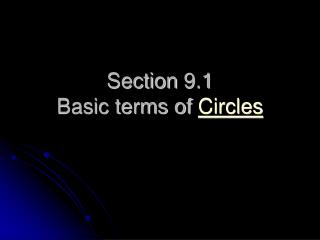# Section 9.1 Basic terms of Circles - PowerPoint PPT PresentationDownload PresentationSection 9.1 Basic terms of Circles

Section 9.1 Basic terms of CirclesDownload Presentation## Section 9.1 Basic terms of Circles

- - - - - - - - - - - - - - - - - - - - - - - - - - - E N D - - - - - - - - - - - - - - - - - - - - - - - - - - -
##### Presentation Transcript

1. Section 9.1Basic terms of Circles

2. What is a circle? • Circle: set of points equidistant from the center • Radius: segment from the center to a point on a circle

3. Chord: segment with endpoints on a circle • Secant: line that contains a chord • Diameter: a chord that contains the center of the circle

4. Tangent Line TANGENT • Tangent: a line that intersects the circle in exactly one point • Point of tangency: the point where the tangent line intersects the circle Point of Tang.

5. Spheres • Sphere: set of all points in space at the same distance from the center

6. Congruent Circles • (or spheres) are circles (or spheres) that have congruent radii

7. Concentric • Concentric circles are circles that lie in the same plane and have the same center. • Concentric spheres are spheres that have the same center

8. Inscribed vs. Circumscribed • Inscribed in a circle means on the inside of the circle. • Try to inscribe a triangle into a circle. • Circumscribed about a square means a circle drawn around a square. • Try to circumscribe a rectangle about a circle.

9. Inscribed vs. Circumscribed • Which shape(s) are inscribed? • Which shape(s) are circumscribed? The square is inscribed in the circle. The circle is inscribed in the triangle. The triangle is circumscribed about the circle but NOT the square. (It must hit the endpoints on the square and it doesn’t.)

10. Copy the following diagram. • Name three radii • Name a diameter • Why is segment TK not a chord? • Name a tangent to circle O. • What name is given to point L. • Consider segment RS to line RS. Which is a chord and which is a secant? Both endpoints aren’t ON the circle Point of Tangency Segment RS is a chord because it has endpoints.

11. Practice Problems • Page 330 W.E. #1, 4, 5, 9 – 15, 18